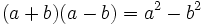# Conjugate

The conjugate is where we change the sign in the middle of two terms like this:We only use it in expressions with two terms, called "binomials":example of a binomial

Here are some more examples:

Expression   Its Conjugate
x2 − 3   ⇒   x2 + 3
a + b   ⇒   a − b
a − b3   ⇒   a + b3

## Examples of Use

The conjugate can be very useful because ...

... when we multiply something by its conjugate we get squares like this:### How does that help?

It can help us move a square root from the bottom of a fraction (the denominator) to the top, or vice versa. Read Rationalizing the Denominator to find out more:

### Example: Move the square root of 2 to the top:13−√2

We can multiply both top and bottom by 3+√2 (the conjugate of 3−√2), which won't change the value of the fraction:

13−√2 × 3+√23+√2 = 3+√232−(√2)2 = 3+√27

(The denominator becomes (a+b)(a−b) = a2 − b2 which simplifies to 9−2=7)

Use your calculator to work out the value before and after ... is it the same?

So try to remember this little trick, it may help you solve an equation one day!